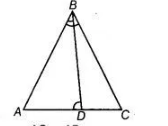# D is any point on side AC of a ΔABC`
Question:

D is any point on side AC of a ΔABC with AB = AC. Show that CD < BD.

Solution:

Given in $\triangle A B C, D$ is any point on side $A C$ such that $A B=A C$. To show $C DC D$.Proof In $\triangle A B C$, $A C=A B$ [qiven]

$\Rightarrow$ $\angle A B C=\angle A C B$ ...(i)

[angles opposite to equal sides are equal]

In $\triangle A B C$ and $\triangle D B C$, $\angle A B C>\angle D B C$ [since, $\angle D B C$ is a internal angle of $\angle B$ ]

$\Rightarrow$ $\angle A C B>\angle D B C$ [from Eq. (i)]

$\Rightarrow$ $B D>C D$ [side opposite to greater angle is longer]

or  \$C D